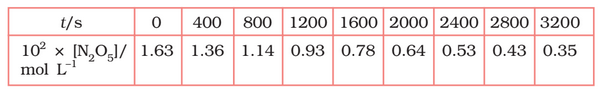# 4.15 (5)   The experimental data for decomposition of  in gas phase at 318K are given below:Calculate the rate constant.

M manish

From the log graph,

the slope of the graph is =
= -k/2.303                             ..(from log equation)

On comparing both the equation we get,

Exams
Articles
Questions